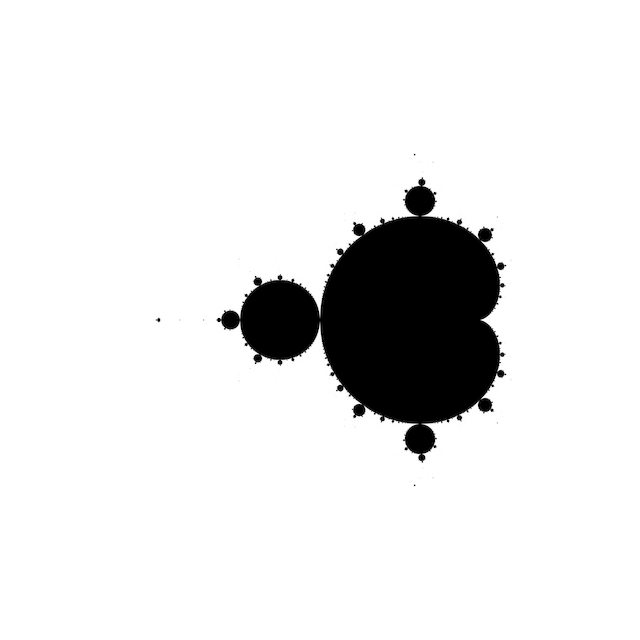# # MembershipIf $$|z|$$ gets large enough, it will keep on getting larger and larger.

Colour according to whether the iterations diverge to infinity or not, within a fixed maximum number of iterations $$N$$ and escape radius $$R \ge 2$$. The disconnected specks visible in the image turn out to be copies of the whole, and despite appearances the Mandelbrot set is connected.

## # 1 C99 Code

#include <complex.h>
#include <stdbool.h>

bool m_membership(int N, double R, double _Complex c)
{
double _Complex z = 0;
for (int n = 0; n < N; ++n)
{
if (cabs(z) > R)
return false;
z = z * z + c;
}
return true;
}